New update is available. Click here to update.
Last Updated: Jun 30, 2023
Easy

# to_string Function in C++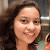Neelakshi Lahiri
0 upvote

## Introduction

Do you like drinking milk? Maybe some chocolate milk will be better?
Now, do you like vanilla ice cream? You’d rather choose chocolate ice cream, wouldn’t you?
A hint of chocolate makes all these different food items more delectable. So, if you’re a person who always has a bottle of chocolate syrup in their fridge, you probably know how important it is to add a hint of chocolate to a couple of your dishes.

Similarly, as a programmer, we may need to change data from one data type to another, particularly easy-to-use ones. For this purpose, there are usually predefined functions in a programming language. The to_string function in C++ is one such function.

But what is it?

Don’t worry. We’ve got you covered. In this article, we will learn all about the to_string function in C++.

Then, what are we waiting for? Let’s go!

Also see, Literals in C.

## What is the to_string function in C++?

From the name “to_string” can you guess what the to_string function in C++ is?

If you guessed it’s a function to convert variables of different data types to string, you are correct!

The to_string function in C++ is a function that returns the string value of integers, decimals, exponents, and expressions after calculation.

Since we know about the to_string function in C++, we must also know its alternatives.

In all, there are four different ways to convert integer data to a string:

(i) StringStream

(ii) to_string function

(iii) Boost.Lexical_Cast

(iv) sprintf( ) function

## Syntax of the to_string function in C++

Knowing what the to_string function in C++ is won’t be helpful until we understand how to use it. For that, we must know the syntax of the to_string function in C++.

The general syntax of the to_string function in C++ is

``STRING VARIABLE = to_string(DATA);``

The “STRING VARIABLE” is a string-type variable that will store the value returned by the to_string function in C++. “DATA” is a variable of any other data type converted to a string.

For a better understanding of the syntax, let us run some code to see how it works.

## Examples of the to_string function in C++

In the definition of the to_string function in C++, we saw that it is a function used to convert integers, decimals, exponents, and expressions after calculation to string.

Let us now see some examples of the actual conversion.

### Integer to String

#### Code

``````#include <iostream>
#include <string>
using namespace std;

int main()
{
// Integer variable
int i=17;

// Integer is converted to a string and stored in a string-type variable
string str=to_string(i);

// In the output, “i” represents an integer
cout<<"Data type of i: "<<typeid(i).name()<<endl;

// The value printed here represents a string value
cout<<"Data type of str: "<<typeid(str).name();

return 0;
}``````

#### Output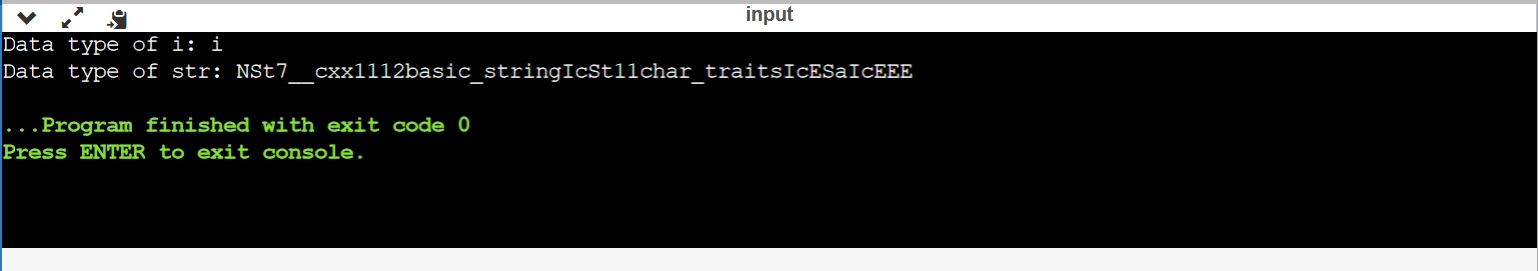### Decimal to String

Decimals may be either float or double. The example below shows the conversion to string for both.

#### Code

``````#include <iostream>
#include <string>
using namespace std;

int main()
{
// Decimal values in double and float variables
double i=17.0;
float j=12.34;

// Double is converted to a string
string str1=to_string(i);

// Float is converted to a string
string str2=to_string(j);

// In the output, “d” represents a double
cout<<"Data type of i: "<<typeid(i).name()<<endl;

// In the output, “f” represents a float
cout<<"Data type of j: "<<typeid(j).name()<<endl;

// The values printed here represent a string value
cout<<"Data type of str1: "<<typeid(str1).name()<<endl;
cout<<"Data type of str2: "<<typeid(str2).name();

return 0;
}``````

#### Output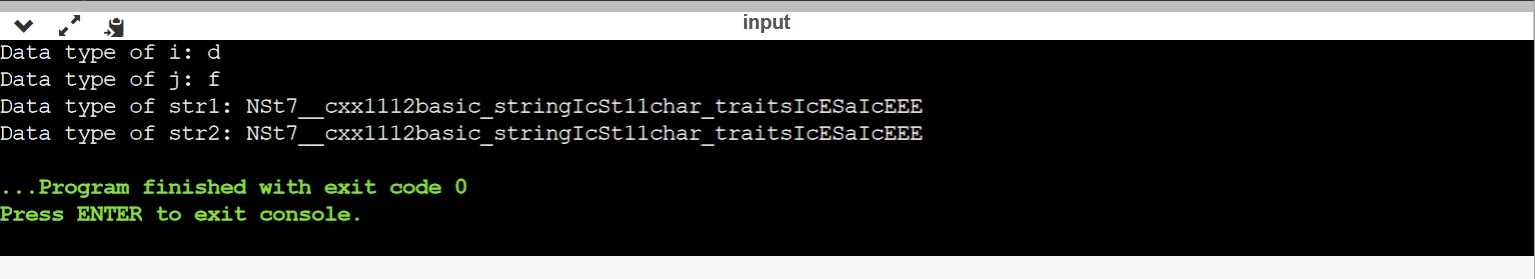### Exponents to String

Exponents are expressed in C++ as double variables using the pow( ) function in math.h.

#### Code

``````#include <iostream>
#include <string>
#include <math.h>
using namespace std;

int main()
{
// Exponential value
double i=pow(2.0,2.0);

// Exponent is converted to a string
string str=to_string(i);

// In the output, “d” represents a double since exponents are stored in a double variable
cout<<"Data type of i: "<<typeid(i).name()<<endl;

// The value printed here represents a string value
cout<<"Data type of str: "<<typeid(str).name()<<endl;

return 0;
}``````

#### Output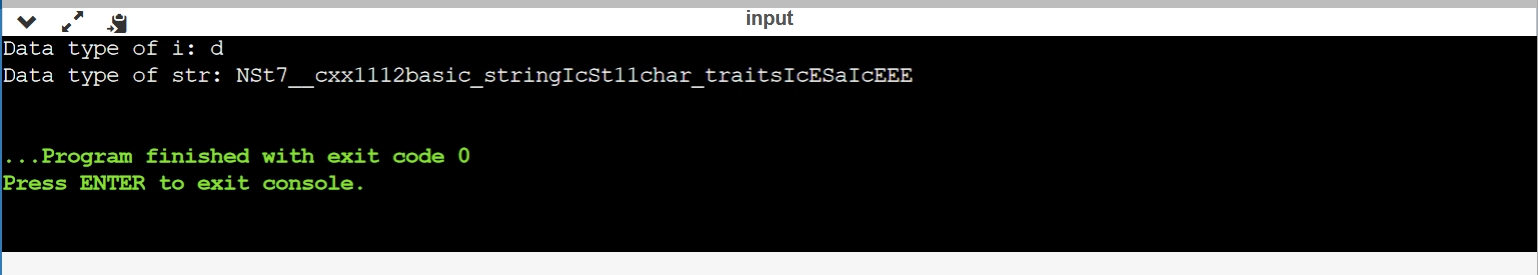### Expressions to String

Expressions in C++ mean any mathematical expression as we know. While converting them, the expression is first evaluated, and then the data type of the result is converted to a string.

#### Code

``````#include <iostream>
#include <string>
#include <math.h>
using namespace std;

int main()
{
// An expression is directly passed to the to_string function and converted to a string
string str=to_string(1+2*3/4-5);

// Since the result of the expression is an integer, so it is stored in a integer variable
cout<<"Data type of the expression: "<<typeid(1+2*3/4-5).name()<<endl;

// The value printed here represents a string value
cout<<"Data type of str: "<<typeid(str).name();

return 0;
}``````

#### Output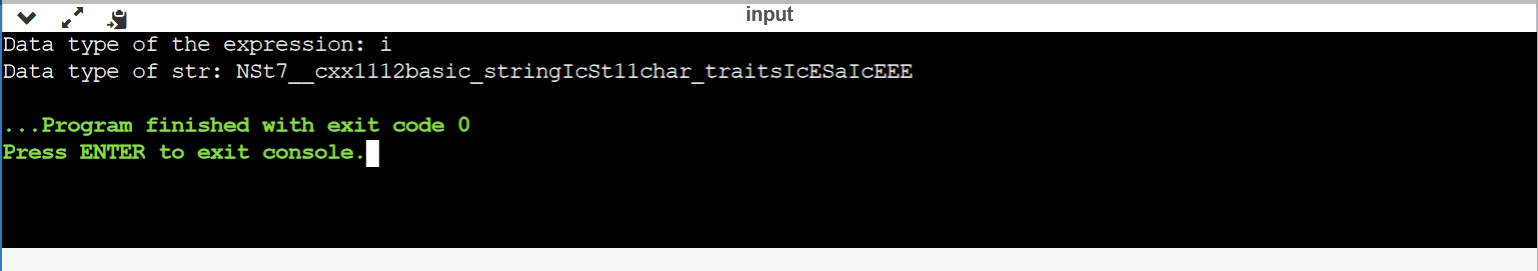Try and compile with online c++ compiler.

With this, we come to the end of our discussion on the to_string function in C++.

Now a question may come to your mind, where do we use the to_string function in C++?

An easy answer to this is while reversing a number. We can easily extract the digits of a number, convert them to a string and concatenate them into another string.

Now, this is a very basic example. We need to practice coding to use the to_string function in C++. For that, you may visit Coding Ninjas Studio by Coding Ninjas.

Let us now see some frequently asked questions.

### What is the to_string function in C++?

The to_string function in C++ is a function that returns the string value of integers, decimals, exponents, and expressions after calculation.

### How can we convert an integer to a string in C++?

The different ways to convert an integer to a string in C++ are StringStream, the to_string function, Boost.Lexical_Cast and the sprintf( ) function.

### How do we use the to_string function in C++?

The syntax to use the to_string function in C++ is STRING VARIABLE = to_string(DATA);.

### What different data types can be converted to strings using the to_string function?

Integers, decimals, exponents, and expressions after calculation can be converted to a string using the to_string function in C++.

### How is an expression converted to a string using the to_string function in C++?

To convert an expression to a string using the to_string function in C++, it is first evaluated and then the data type of the result is converted to a string.

## Conclusion

Programming is a concept just like maths. Just like we have different operations in maths, we have different functions in programming.

In this article, we saw one such function - the to_string function in C++. We learned how to use it, where it is used, and even saw some examples.

Other than the to_string function in C++, there are many other functions in C++ we should learn about too. Some of them are:

Apart from that, don’t forget to check out Data Structures and AlgorithmsCompetitive ProgrammingBasics of C++Data Structures and Algorithms in C++, and many more courses on Coding Ninjas.

Happy coding!